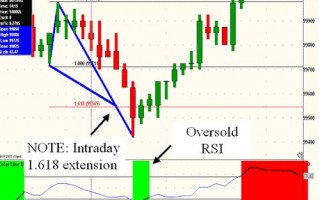# Forex strategies for RSI and Fibonacci extension 1.618

Forex strategies, based on the method of harmonious trade based on Fibonacci ratios 1.618 and forex indicator RSI.

Let us consider the simple technique of Fibonacci extension 1.618 to the price movement on the chart in conjunction with the indicator Forex Relative Strength Index — RSI (levels 30 and 70), which should first be installed on a timetable of the trading terminal MetaTrader 4.

## Fibonacci ratio 1.618

The projection of 1.618 Fibonacci ratio is significant, because it often indicates the depletion of price movement. Once the market is higher than the 1.618 Fibonacci extension, it often represents an extreme price action, which is very difficult to maintain for a long time. Once the design of 1.618 was achieved, this means that in this area of the graph formed trading opportunity for entry into the market. Since this level of Fibonacci 1.618 represents the price action, which is extreme and it is in this area may be the rapid emergence of the trend reversal in the market.

Relative Strength Index — RSI

As you probably already know from the technical analysis of Forex — Relative Strength Index (RSI) is sufficiently important oscillator, followed by the price, which fluctuates between the values 0 and 100. Forex Relative Strength Indicator RSI often rises above the level of 70 and falls below 30 that conform to overbought and oversold levels respectively.

Bullish design Fibonacci level 1.618

Bullish design Fibonacci level 1.618 is very often happens in an emergency sale on the market. As soon as the market declines, the price action tends to often become oversold. And that’s the point of designing Fibonacci 1.618 tends to give the trader a profitable opportunity for a deal on the market, because market as soon as the unfolding of this area, mainly provides a good strong trend.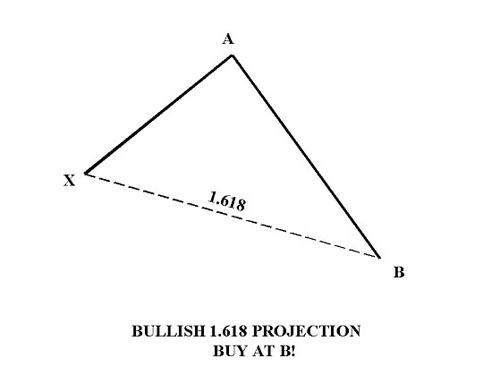Bullish design Fibonacci level 1.618

Let’s look at Example 1, as after massive sale on the market formed an excellent intra-day extension of the Fibonacci 1.618.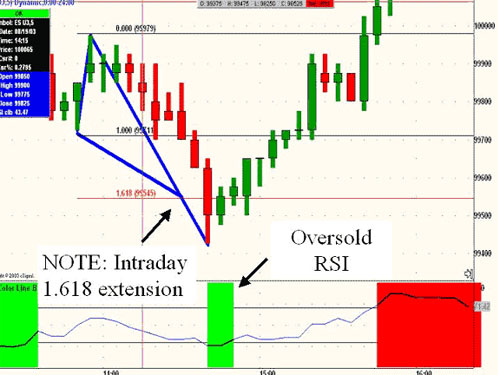Example 1

It should be noted that preferred to use only the Fibonacci extension 1.618, which are formed during active market hours of the trading day (9:30 — 16:00 ET). In this case, the early sale provided a good spread in the market, and this was in turn confirmed the value of indicator Forex oversold RSI, which was below the oversold 30.

Bear designing Fibonacci 1.618

Bear designing Fibonacci 1.618 is highly significant Fibonacci ratio, because is an excellent area to take profits or to make a deal to sell (short position). This level of Fibonacci extension 1.618 represents extreme price area in which very often occur trend reversals or setbacks. Once the price reaches a given level of Fibonacci, it faces considerable resistance in the market. And if it so happens, the direction of movement in the market often reverses.

Although the area Fibonacci 1.618 may be slightly exceeded. But while it is important to understand that such a movement above this area Fibonacci would be difficult to maintain for a long time. Fibonacci level 1.618 in combination with indicator forex RSI is an extremely effective tool for finding good deals. Therefore, it is important to closely monitor the behavior of the market in this area and always be ready to open trading positions for sale.

## Let’s look at another example:

Relative Strength Index RSI affirmed the short-term resistance. After a sharp morning rally, the index stood at ES Fibonacci extension 1.618 at around 992.75 and the indicator forex RSI have an overbought condition (while being above the level 70). The market then turned around at 993.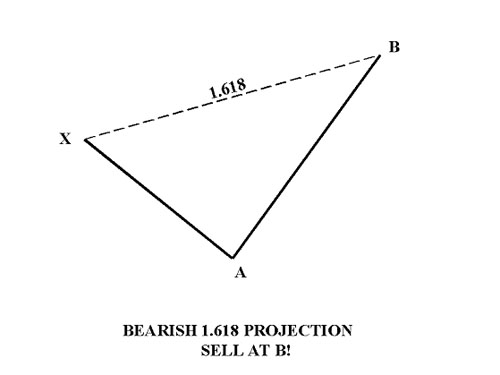Bear designing Fibonacci 1.618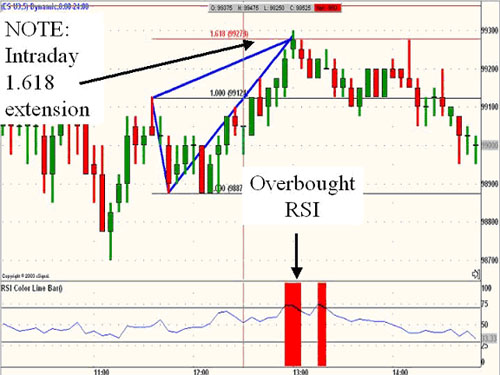Example 2

It is important to note in this example that the RSI indicator has provided dual meaning overbought level above 70 and RSI divergence is formed within 20 minutes, thus providing further evidence of resistance within the harmonic-day Fibonacci extension 1.618.

Bargaining Strategy Forex RSI and Fibonacci extension 1.618:

Bargain on the forex strategy should either limit orders set at the level of 1.618 on the selected motion or after a rebound from this Fibonacci level and at the opening of the next hour, just to draw attention to the indicator RSI (where he is — in the oversold or overbought) and only then open trading position.

But do not forget those not menie about stop-loss orders and repositioning to zero. It is better to use the same standard trailing stop (the size depends on the chosen currency pair and time period).